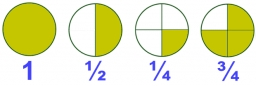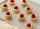# Product increased

When the product of 2/3 and 6/10 is increased by 2/5, the result is?

x =  0.8

### Step-by-step explanation:Did you find an error or inaccuracy? Feel free to write us. Thank you!Tips to related online calculators
Need help to calculate sum, simplify or multiply fractions? Try our fraction calculator.

## Related math problems and questions:

• Quotient and productIf the quotient of [8/5 divided by 8/10] is added to the product of [8/14 x 7/12 x 3/8], what is the sum?
• Two numbers and its productThe product of two numbers are 2/3. If on of them is 1/10, what is the other?
• FractionsSort fractions z1 = (6)/(11); z2 = (10)/(21); z3 = (19)/(22) by its size. Result write as three serial numbers 1,2,3.3 3/4 + 2 3/5 + 5 1/2 Show your solution.
• Evaluate fractionsThe difference  of 5 1/2 and 2/3 is added to the product of 5/6 and 1/2
• Evaluate 11Multiply the quotient of 6 and 2 by 3, then add 1. Add 1 and 6, then divide by 2 and multiply by 3. Divide 6 by the product of 2 and 3, then add 1.
• Expression plus minusEvaluate expression: (-1)2 . 12 – 6 : 3 + (-3) . (-2) + 22 – (-3) . 2
• Length subtractingExpress in mm: 5 3/10 cm - 2/5 mm
• Fractions and mixed numerals(a) Convert the following mixed numbers to improper fractions. i. 3 5/8 ii. 7 7/6 (b) Convert the following improper fraction to mixed number. i. 13/4 ii. 78/5 (c) Simplify these fractions to their lowest terms. i. 36/42 ii. 27/45 2. evaluate following ex
• Simplify 7Simplify. 7-1/3÷ 3-2/3 of 2+ 4-1/2÷ 2-1/4+ 1/2 solution and by step by stepWhy does 1 3/4 + 2 9/10 equal 4.65? How do you solve this?What must be subtracted from the sum of 3/8 and 5/16 to get difference equal to the product of 5/8 and 3/16?What is the sum of 2/3 and 3/10?Add two mixed fractions: 2 4/6 + 1 3/6After a special event, a caterer examined the leftovers on the serving table. She saw 10/11 of a tartlet with apples, 2/3 of a tartlet with strawberries, and 10/11 of a tartlet with raspberries. How many leftover tartlets did the caterer have?Calculate the product of the sum and difference of numbers -7 and -2.When (7¹/3 + 2¾)÷(2 + 2¼ × 1¹/3) is simplified the result is?